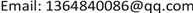1. 引言

2. 基于线性回归未完成任务分析2.1. 任务空间分布分析

E = − 1.2827 N + 142.9765 (1)

d max = 0.940944 ,                                 d min = 0.000495

p max = 85 , p min = 65

p i = p min + ( p max − p min ) ( d i − d min ) d max − d min

p i = 21.27 d i + 65

1) 无论是完成度为1还是完成度为0的任务，其定价都与完成度没有明显的关联。这也从侧面验证了我们什么对于“定价仅与地理位置有关”的猜想是正确的。

2) 完成度为1的任务点相对集中在中间区域，而完成度为0的区域相对集中在两端。因此我们猜想完成度与地理位置有一定的关联。同时也说明了原先的定价方案对于地域因素考虑的还不够全面。

3) 广州和深圳地区的任务完成度整体较低，而东莞、佛山等地的任务完成度较高。所以可能是在定价的时候忽略了不同地区的经济情况，从而导致定价不合理，部分任务完成度偏低  。

2.2. 任务价格的修缮

2.3. 价格制定的完善

q i = q min + ( q max − q min ) ( t i − t min ) t max − t min (4)

q max = 85 , q min = 65 , t min = 3.8044 , t max = 10863.66

q i = 0.00184 t i + 65 (5)

p i = 21.27 d i + 65 (3)

Q ( p i , q i ) = λ p i + ( 1 − λ ) q i

λ characteristic value tabl
λ0.10.20.30.40.50.60.70.80.9
ni522558511502485479476456444
ωi0.6120.6540.5990.5890.5690.5620.5580.5350.521

Q = 4.254 d + 0.001472 t + 65 (6)

3. 打包任务后价格的模型3.1. 打包标准

1) 两打包点之间的距离短。(绝对距离短)

2) 打包点之间的距离比到用户之间的距离短  。(相对距离短)

3.1.1. 打包点的确立

E ( D w ) = 0.02548 , Z ( D w ) = 0.01325

D w < Z (Dw)

| A 1 A 2 | < | A 1 B | ,             | A 1 A 2 | < | A 2 B |

3.1.2. 关于打包后任务的定价方案

Packing price lis
A1A2B1B2C1C2

d0.102230.37130.07370.18830.50220.00189

4. 结语

1) 模型打包之后，任务可以更快地交付给会员们，有效提高了任务完成效率，也可以提高会员的积极性。

2) 主成分分析法是通过考虑多个因素来寻找对某一种变量的影响，具有很好的应用性，在众多相似问题中都可以用这类打包方法，比如快递跑腿，外卖配送软件、图像处理等方面。

3) 结合了SPSS，CODEBLOCKS，MAT LAB等软件，灵活地运用主成分分析法，曲线拟合等方法，使结论更加精确。